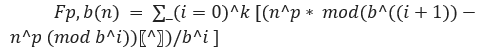# Dudeney Numbers in C++

C++Server Side ProgrammingProgramming

A mathematical number defined in number theory in a given number base is a natural number equal to the perfect cube of another natural number such that the digit sum of the first natural number is equal to the digit sum of the second number(wikipedia).

The number was found by Henry Dudeney. Its mathematical formula is −Here, we are given an integer n. Our task is to check whether the given number n is a dudeney number or not.

## Let’s take an example to understand the problem,

Input: N = 17592

Output: No

Explanation:

The given number is not a dudney number.

## Solution Approach −

The solution lies in the basic definition of dudeney number. A number is a dudeney number based on the fact that the cube root of a number is equal to the sum of its digits.

## Algorithm −

Step 1: Check if n is a perfect cube.

Step 2.1: If YES, then check if the cube root of n = sum of digits of n.

Step 2.2.1: If YES, then the number is Dudeney number.

Step 2.2.2: If NO, then the number is not a Dudeney number.

Step 2.2: If NO, then the number is not a Dudeney number.

## Example

Live Demo

#include <bits/stdc++.h>
using namespace std;

int calcDigitSum(int n){

int digitSum = 0;
int digitVal;
while (n > 0) {
digitVal = n % 10;
digitSum += digitVal;
n /= 10;
}
return digitSum;

}
int checkDudeney(int N) {

int cubeRoot = int( round( cbrt(N) ) );

if(pow(cubeRoot, 3.0) != N){
return 0;
}

int sumOfDigit = calcDigitSum(N);

if (cubeRoot != sumOfDigit)
return 0;

return 1;
}

int main() {
int N = 104323;
cout<<"The number "<<N;
if (checkDudeney(N))
cout<<" is a dudeney number.";
else
cout<<" is not a dudeney number.";
return 0;
}

## Output −

The number 104323 is not a dudeney number.